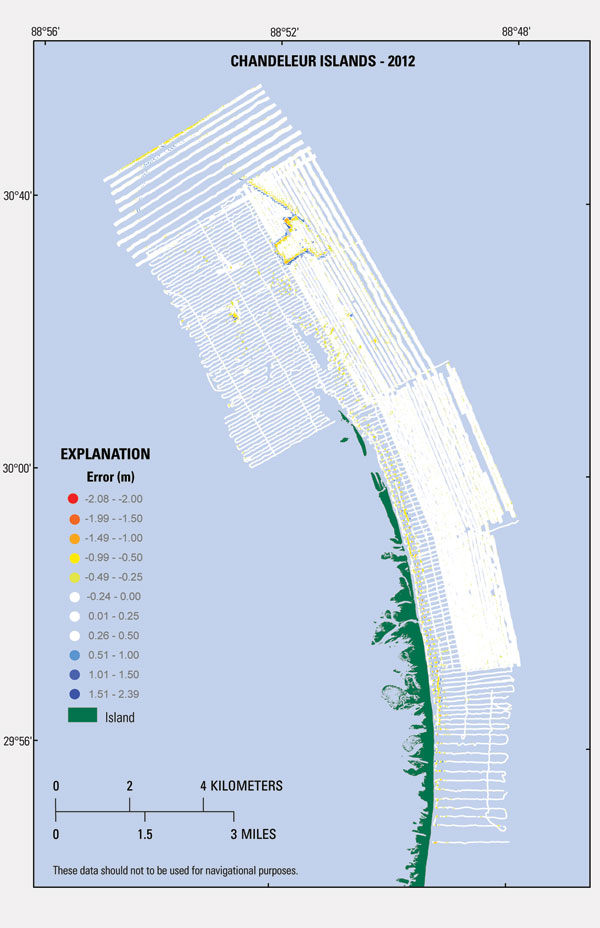Data Series 847

### Coastal Bathymetry and Backscatter Data Collected in 2012 from the Chandeleur Islands, Louisiana

 Index Abstract Introduction Survey Overview and acquisition Data Processing Error Analysis Survey Products Data Downloads Logs Acronyms References Cited

## Error Analysis

Single Beam

The single-beam data were imported into ArcGIS, and a locally produced Python script was created to evaluate elevation differences at the intersection of crossing tracklines. The script calculates the elevation difference between points at each intersection using an inverse distance weighting equation. Elevation values at line crossings should not differ by more than the combined instrument acquisition error (per manufacturer specified accuracies, tables 1 and 2). GPS cycle slips, stormy weather conditions, and rough sea surface states can contribute to poor data quality. If discrepancies that exceed the acceptable error threshold were found, then the linein error was either statically adjusted or removed. A line in error constitutes one or more of the following: (1) a segment where several crossings are incomparable, (2) a known equipment problem, or (3) known bad GPS data identified in the post-processing steps. For this dataset, elevation differences at all crossings were less than or equal to 0.15 m, and the elevation differences were within +/- 0.10 m for 89 percent of the 653 crossings at which the horizontal distance between soundings was less than 2 m.

Interferometric Swath

The stated horizontal accuracy of the OmniSTAR HP navigation subscription used during swath bathymetry acquisition is +/- 10 centimeters (cm) horizontally and +/-15 cm vertically. The Coda Octopus F190R IMU integrates the OmniSTAR HP position with motion, measures vessel velocity (+/- 0.014 m/s), roll (+/- 0.025 degrees), pitch(+/- 0.025 degrees), heading (1 m baseline  0.1 degrees), and heave (5 cm per meter of depth) (table 3). The vertical accuracy for the SWATHplus-H system varies with depth and across track range. At 57 m it is accurate to 10 cm vertically (table 4). Sound velocity captured in real-time at the transducer head was collected by a Valeport Mini SVS with a stated maximum error of +/- 0.017 m/s (table 5). Sound velocity profile cast data tables were also applied to the swath data based on time, water depth, and spatial representation during the survey. The SVP data was captured with a Valeport Mini SVP with stated accuracy of +/- 0.02 m/s (table 6).

Vertical Transformation

In addition to positional accuracies inherent for different datums (for example, NAD83, σ = 2.0 cm; NAVD88, σ = 5.0 cm; Mean Lower Low Water, MLLW, σ = 1.6 cm), error is introduced every time the data is transformed between datums. The transformation from ellipsoid height (ITRFXX) to orthometric height (NAVD88) is σ = 7.0 cm, and the maximum cumulative uncertainty (quantified by standard deviation) introduced when transforming from ellipsoid height to orthometric height to a tidal datum (for example, MLLW) is 17.1 cm for eastern Louisiana (http://vdatum.noaa.gov/docs/est_uncertainties.html).

Digital Elevation Model

Point data from the interferometric swath system (samples from the 5-m BASE surface nodes) and single-beam soundings were merged into a single x,y,z dataset. Using the Spatial Analyst tool in Esri ArcGIS, the soundings were compared to the final combined DEM by extracting values from the DEM at the precise sounding sample locations used to create the model. There are a total of 2,406,226 samples and the root mean square error of all points is 0.166 m. The root mean square error of 91 percent of all points is 0.098 m. The differences between the point dataset and the extracted DEM values were color coded in order to visually articulate differences (fig. 7). This comparison demonstrates how well the model surface represents the original sounding and sample data. The interpolated DEM resolution was constrained by a 50-m cell size and the distance between tracklines was generally in excess of 150 m (fig. 5).

Depending on how coarse the DEM resolution is compared to sounding distribution greatly effects how well the DEM represents the data. In this example there may be hundreds of samples in a 50-m by 50-m cell; however, they are only represented on the surface by one value, typically the mean. Therefore, in cases where terrain slope is high the gridding formula mutes the actual variability in the sample data. This sacrifice is necessary when creating continuous surfaces over bathymetric datasets where trackline spacing did not allow for overlap. Finlayson and others (2011), Fregoso and others (2008), and Foxgrover and others (2004) also reported difficulty with gridding over steep slopes.The percent coverage of swath sample data relative to the elevation model area is approximately 80.25 percent, encompassing 81 km2.Figure 7. Error values representing the difference between the bathymetry grid (DEM) at each sample point. White symbols represent acceptable system error of +/-0.240 meters. Island area extent is derived from USGS lidar data collected in February 2012. [Click to enlarge.]U.S. Department of the Interior | U.S. Geological Survey
URL: https://pubs.usgs.gov/ds/0847/ds847_error.html